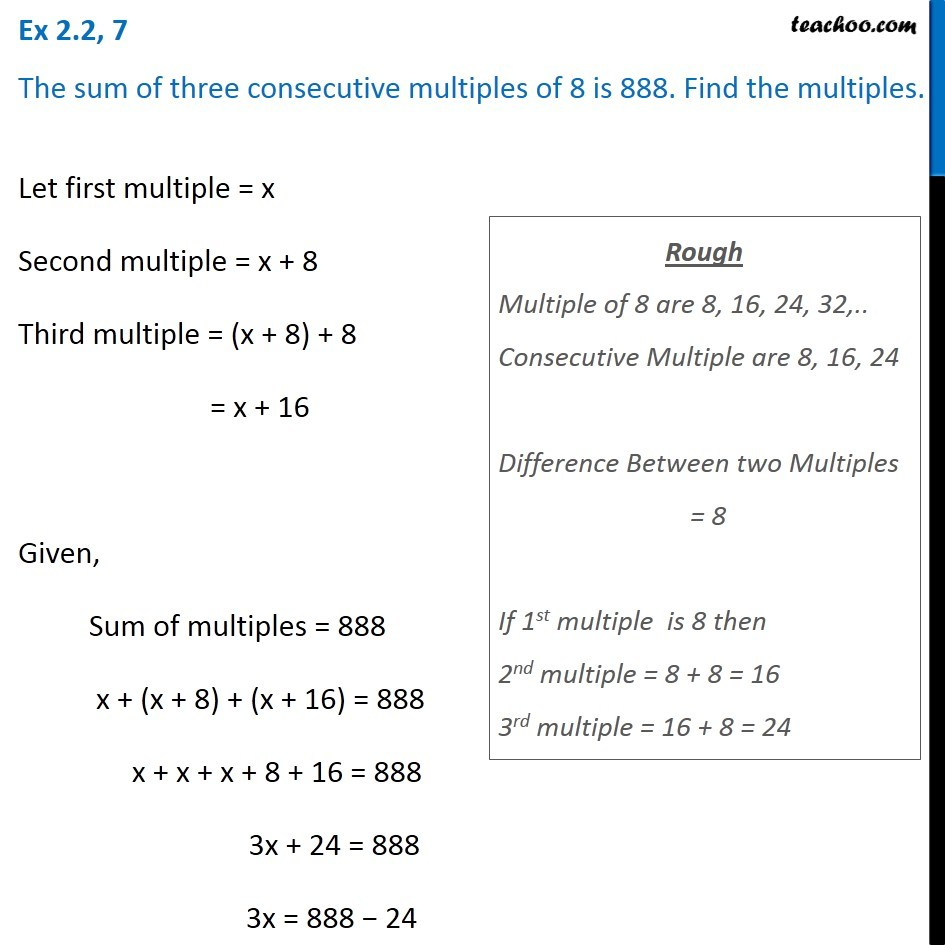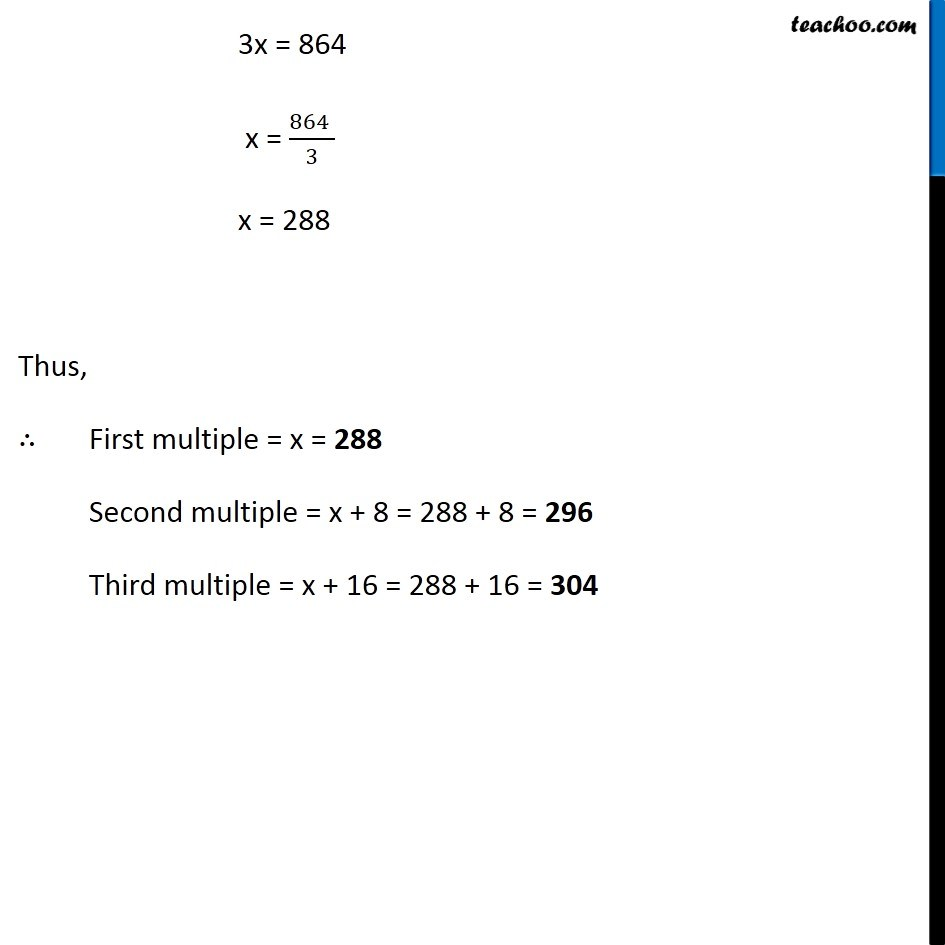Solving easy equations - Word Problems

Chapter 2 Class 8 Linear Equations in One Variable
Serial order wiseLearn in your speed, with individual attention - Teachoo Maths 1-on-1 Class

### Transcript

Question 7 The sum of three consecutive multiples of 8 is 888. Find the multiples.Let first multiple = x Second multiple = x + 8 Third multiple = (x + 8) + 8 = x + 16 Given, Sum of multiples = 888 x + (x + 8) + (x + 16) = 888 x + x + x + 8 + 16 = 888 3x + 24 = 888 3x = 888 − 24 Rough Multiple of 8 are 8, 16, 24, 32,.. Consecutive Multiple are 8, 16, 24 Difference Between two Multiples = 8 If 1st multiple is 8 then 2nd multiple = 8 + 8 = 16 3rd multiple = 16 + 8 = 24 3x = 864 x = (864 )/3 x = 288 Thus, ∴ First multiple = x = 288 Second multiple = x + 8 = 288 + 8 = 296 Third multiple = x + 16 = 288 + 16 = 304